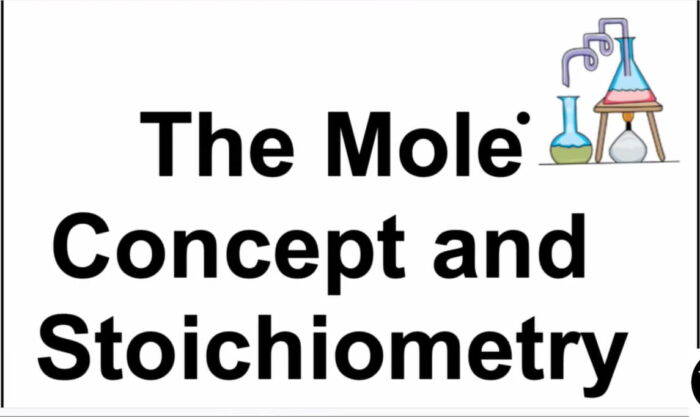Stoichiometry of redox reactions means calculations of the quantities of the oxidizing and reducing agents and their products in oxidation reduction reactions. Redox, on the other hand, is a type of chemical reaction in which the oxidation states of substrate change. Oxidation is the loss of electrons or an increase in the oxidation state, likewise, reduction is the gain of electrons or a decrease in the oxidation state.

## Course Content

• Redox processes: electron transfer and changes in oxidation number (oxidation state)
• Relative masses of atoms and molecules
• The mole, the Avogadro constant
• The calculation of empirical and molecular formulae
• Reacting masses and volumes (of solutions and gases)

## Learning Outcomes

• the term relative formula mass or M r will be used for ionic compounds

Candidates should be able to:

• (a) define the terms relative atomic, isotopic, molecular and formula mass
• (b) define the term mole in terms of the Avogadro constant
• (c) calculate the relative atomic mass of an element given the relative abundances of its isotopes
• (d) define the terms empirical and molecular formula
• (e) calculate empirical and molecular formulae using combustion data or composition by mass
• (f) write and/or construct balanced equations
• (g) perform calculations, including use of the mole concept, involving:
• (i) reacting masses (from formulae and equations)
• (ii) volumes of gases (e.g. in the burning of hydrocarbons)
• (iii) volumes and concentrations of solutions
(when performing calculations, candidates’ answers should reflect the number of significant figures given or asked for in the question)
• (h) deduce stoichiometric relationships from calculations such as those in (g)